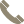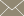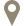Home » Faculty Profiles » Faculty

### Hussain, Saqib

Assistant Professor

#### CONTACT INFO(956) 326-2570saqib.hussain@tamiu.eduLBV-321

#### COURSES TAUGHT

• Abstract Algebra I (MATH 4310)
• Abstract Algebra I (MATH 5330)
• Advanced Linear Algebra (MATH 3318)
• Applied Multivariate Analysis (STAT 5341)
• Business Math I (MATH 1324)
• Business Math II (MATH 1325)
• Calculus I (MATH 2413)
• Calculus III (MATH 2415)
• College Algebra (MATH 1314)
• Discrete Mathematics (MATH 3365)
• Functional Analysis I (MATH 5312)
• Generalized Lin Mdls with Apps (STAT 5306)
• Introduction to Topology (MATH 4360)
• Introductory Statistics (MATH 1342)
• Numerical Analysis II (MATH 4341)
• Numerical Linear Algebra (MATH 4330)
• Numerical Methods for PDE I (MATH 5367)
• Ordinary Diff Equations (MATH 3330)
• Pre-Calculus (MATH 2412)
• Probability (MATH 5375)
• Quality Control & Improvement (STAT 5340)
• Reg & App Time Series Mdls (STAT 5328)
• Theory of Sampling & Surveys (STAT 5322)
• Thesis I (MATH 5398)
• Thesis II (MATH 5399)